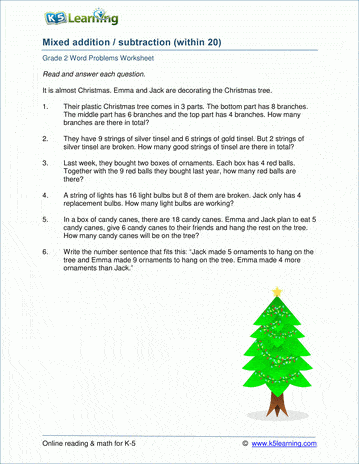# Math Word Problems Worksheets

## Word problems worksheets for kindergarten to grade 5

Our word problems worksheets are best attempted after a student is familiar with the underlying skill. We include many mixed word problems or word problems with irrelevant data so that students must think about the problem carefully rather than just apply a formulaic solution.

Kindergarten:

Topics include:

• Simple word problems with 1-digit addition

## Kindergarten subtraction word problems

• Simple word problems with 1-digit subtraction

## Grade 1 word problems worksheets

• Single digit addition word problems
• Addition with sums 50 or less
• Adding 3 or more numbers
• Subtracting 1-digit numbers
• Subtracting numbers under 50
• Time and elapsed time
• Counting money word problems
• Measurement word problems (lengths)
• Writing fractions from a story
• Mixed word problems

## Grade 2 word problems worksheets

• 1,2 and 3-digit addition word problems
• 1,2 and 3-digit subtraction
• Multiplication within 25
• Lengths - adding / subtracting / comparing (customary and metric)
• Time and elapsed time (1/2 hour intervals)
• Time and elapsed time (5 minute intervals)
• Counting money (coins and bills)
• Writing fractions word problems
• Comparing fractions

## Grade 3 word problems worksheets

• Simple addition word problems (numbers under 100)
• Addition in columns (numbers under 1,000)
• Mental subtraction
• Subtraction in columns (2-3 digits)
• Simple multiplication (1-digit by 1 or 2-digit)
• Multiplying multiples of 10
• Multiplication in columns
• Simple division
• Long division with remainders (numbers 1-100)
• Mixed multiplication and division word problems
• Identifying, comparing and simplifying fractions
• Adding and subtracting fractions (like denominators)
• Length word problems
• Time word problems (nearest 1 minute)
• Mass and weight word problems
• Volume and capacity word problems
• Word problems with variables
• Mixed word problems

## Grade 4 word problems worksheets

• Four operations (addition, subtraction, multiplication, division)
• Estimating and rounding
• Writing and comparing fractions
• Adding and subtracting fractions (like denominators)
• Multiplying fractions by whole numbers
• Adding and subtracting decimals (up to 3 terms)
• Length word problems (customary and metric units)
• Mass and weight word problems
• Volume and capacity word problems
• Time word problems (including am vs pm)
• Money word problems (with decimal notation)
• Shopping word problems
• Mixed word problems

## Grade 5 word problems worksheets

• Mixed 4 operations (addition, subtraction, multiplication, division)
• Estimating and rounding word problems (based on the 4 operations)
• Add and subtract fractions and mixed numbers (like and unlike denominators)
• Multiplying and dividing fractions
• Mixed operations with fractions (add, subtract, multiply, divide)
• Decimals word problems (add, subtract, multiply)
• Length word problems (customary and metric units)
• Mass and weight word problems (oz, lbs / gm, kg)
• Volume and capacity word problems
• Variables and expressions word problems
• Variables and equations word problems
• Volume of rectangular prism
• GCF / LCM word problems
• Mixed word problems

## Related topics

Fractions worksheets

Geometry worksheetsSample Word Problems Worksheet

What is K5?

K5 Learning offers free worksheets, flashcards and inexpensive workbooks for kids in kindergarten to grade 5. Become a member to access additional content and skip ads.